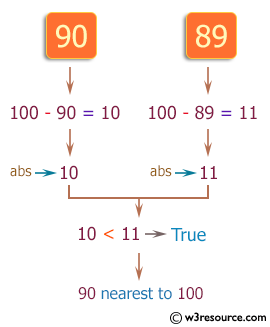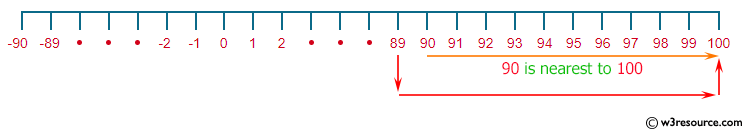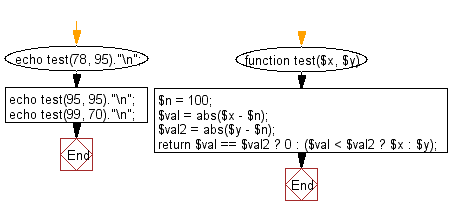﻿ PHP Exercises: Check which number nearest to the value 100 among two given integers - w3resource# PHP Exercises: Check which number nearest to the value 100 among two given integers

## PHP Basic Algorithm: Exercise-19 with Solution

Write a PHP program to check which number nearest to the value 100 among two given integers. Return 0 if the two numbers are equal.

Sample Solution:

PHP Code :

``````<?php
function test(\$x, \$y)
{
\$n = 100;
\$val = abs(\$x - \$n);
\$val2 = abs(\$y - \$n);
return \$val == \$val2 ? 0 : (\$val < \$val2 ? \$x : \$y);
}

echo test(78, 95)."\n";
echo test(95, 95)."\n";
echo test(99, 70)."\n";
``````

Sample Output:

```95
0
99
```

Pictorial Presentation:Flowchart:PHP Code Editor:

What is the difficulty level of this exercise?

﻿

## PHP: Tips of the Day

\$_REQUEST: This SuperGlobal Variable is used to collect data submitted by a HTML Form

Example:

This code save in a php file and run in the browser.

```<!DOCTYPE html>
<html>
<body>

<form method="post" action="<?php echo \$_SERVER['PHP_SELF'];?>">
NAME: <input type="text" name="user">
<button type="submit">SUBMIT</button>
</form>
<?php
if (\$_SERVER["REQUEST_METHOD"] == "POST") {
\$name = htmlspecialchars(\$_REQUEST['user']);
if(empty(\$name)){
echo "Name is empty";
} else {
echo \$name;
}
}
?>
</body>
</html>
```

Output:

```Owen
```